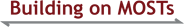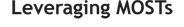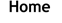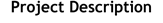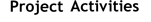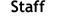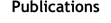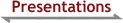Using a Public Record to Anchor Whole-Class Mathematical Discussions

Van Zoest, L. R., Madis, C. E., Leatham, K. R., Peterson, B. E., & Stockero, S. L. (2021, February). Using a Public Record to Anchor Whole-Class Mathematical Discussion. Presentation at the 25th Annual Conference of the Association of Mathematics Teacher Educators, Held Virtually.

Establishing Student Mathematical Thinking as an Object of Class Discussion

Peterson, B. E., Stockero, S. L., Van Zoest, L. R., & Leatham, K. R. (2021, February). Establishing Student Mathematical Thinking as an Object of Class Discussion. Presentation at the 25th Annual Conference of the Association of Mathematics Teacher Educators, Held Virtually.

Public Record: Cornerstone of Productive Whole-Class Mathematical Discussions

Van Zoest, L., Madis, C., & Jokela, S. (2020, April). Public record: cornerstone of productive whole-class mathematical discussions. Presentation at the 100th Annual Meeting of the National Council of Teachers of Mathematics, Chicago, IL.

Let's find ways to improve mathematical discourse! Learn about different uses of a public record to anchor whole-class discussion, see examples of revising a public record in real-time to support the discussion, hear about how intentional use of a public record can improve instruction, and practice some key takeaways for your own classroom.

Learning a Teaching Practice Through Representations, Decompositions, and Approximations

Madis, C., Ruk, J., Van Zoest, L. R., Peterson, B., Stockero, S. & Leatham, K. (2020, February). Learning a teaching practice through representations, decompositions, and approximations. Presentation at the 24th Annual Conference of the Association of Mathematics Teacher Educators, Phoenix, AZ.

Understanding and Developing Skills Needed to Build on Student Mathematical Thinking

Leatham, K. R., Stockero, S. L., Peterson, B. E., & Van Zoest, L. R., (2020, February). Understanding and developing skills needed to build on student mathematical thinking. Presentation at the 24th Annual Conference of the Association of Mathematics Teacher Educators, Phoenix, AZ.

We will discuss the teaching practice of building on student mathematical thinking, unpacking important nuances of this practice. Together we will consider how we as mathematics teacher educators can help teachers to develop skills related to these nuances.

An Elaboration of Four Subpractices of the Teaching Practice of Building on Student Thinking

Leatham, K. R., Stockero, S. L., Peterson, B. E., Van Zoest, L. R., Heninger, A., Seiwell, A., Hollenbeck, C., & Ruk, J. (2019, November). An elaboration of four subpractices of the teaching practice of building on student thinking. Poster presented at the 41st Annual Meeting of the North American Chapter of the International Group for the Psychology of Mathematics Education, St. Louis, MO.

Building: A Way to Productively use High-Leverage Student Mathematical Thinking

Peterson, B. E., Leatham K. R. with Stockero, S. L. & Van Zoest, L. R. (2019, October). Building: a way to productively use high-leverage student mathematical thinking. Presentation at the Regional Conference of the National Council of Teachers of Mathematics, Salt Lake City, UT.

Explore with us ways to productively use student mathematical thinking during instruction—how to take advantage of teachable moments. We will describe four subpractices that comprise the teaching practice of building (make precise, grapple toss, orchestrate, make explicit), discuss what it might look like to enact them well.

Teachers' Initial Responses to High-Leverage Instances of Student Mathematical Thinking

Stockero, S. L., Van Zoest, L. R., Ochieng, M. A., Ruk, J. M., Peterson, B. E., & Leatham, K. R. (2019, July). Teachers' initial responses to high-leverage instances of student mathematical thinking. Presentation at the 43rd Annual Conference of the International Group for the Psychology of Mathematics Education, Pretoria, South Africa.

To Pursue or Not to Pursue: Making Decisions about Student Mathematical Thinking

Peterson, B. E., Leatham, K. R., Van Zoest, L. R., & Stockero, S. L. (2019, April). To pursue or not to pursue: Making decisions about student mathematical thinking. Presentation at the 2019 National Council of Teachers of Mathematics Conference, San Diego, CA.

Incorporating student mathematical thinking into classroom instruction is a best practice, but not all student thinking provides the same leverage for accomplishing mathematical goals. Learn about characteristics of student thinking that can be used to determine which thinking has significant potential to support students' learning of mathematics.

Examining which Student Thinking is Considered in Responsive Teaching

Stockero, S. L., Leatham, K. R., Peterson, B. E., & Van Zoest, L. R. (2019, February). Examining which student thinking is considered in responsive teaching. Presentation at the 23rd Annual Meeting of the Association of Mathematics Teachers Educators, Orlando, FL.

Teachers' Responses to Instances of Student Mathematical Thinking with Varied Potential to Support Student Learning

Stockero, S. L., Freeburn, B., Van Zoest, L. R., Peterson, B. E., & Leatham, K. R. (2018, November). Teachers' responses to instances of student mathematical thinking with varied potential to support student learning. Presentation at the 40th Annual Meeting of the North American Chapter of the International Group of the Psychology of Mathematics Education, Greenville, SC.

Investigating Productive Use of High-Leverage Student Mathematical Thinking

Peterson, B. (2018, June). Investigating productive use of high-leverage student mathematical thinking. Poster presented at the 2018 NSF Discovery Research PreK-12 PI Meeting. Washington, DC.

This project focuses on the teaching practice of building on student thinking, a practice in which teachers engage students in making sense of their peers' mathematical ideas in ways that help the whole class move forward in their mathematical understanding. The study examines how teachers incorporate this practice into mathematics discussions in secondary classrooms by designing tasks that generate opportunities for teachers to build on students' thinking and by studying teachers' orchestration of whole class discussions around student responses to these tasks.

A Characterization of Student Mathematical Thinking That Emerges During Whole-Class Instruction: An Exploratory Study

Van Zoest, L. R., Leatham, K. R., Arslan, O., Ochieng, M. A., Ruk, J. M., Peterson, B. E., & Stockero, S. L. (2018, February). A characterization of student mathematical thinking that emerges during whole-class instruction: An exploratory study. Presentation at the 40th Annual Meeting of the North American Chapter of the International Group for the Psychology of Mathematics Education, Greenville, SC.

This exploratory study investigated 164 instances of student mathematical thinking that emerged during whole-class instruction in a high-school geometry course. The MOST Analytic Framework provided a way to categorize these instances according to their Building Potential—that is, the potential for learning to occur if the student thinking of the instance were made the object of consideration by the class. We discuss variations in Building Potential found in these instances by examining the subcategorizations that emerged from our additional analyses. The variations in the building potential of student thinking revealed in the study highlight the complexity of teaching, and the need to support teachers in identifying and appropriately responding to instances with different levels of Building Potential.

Teachers' Orientations Around Using Student Mathematical Thinking as a Resource During Whole-class Discussion

Leatham, K. R., Stockero, S. L., Ochieng, M. A., Van Zoest, L. R., & Peterson, B. E. (2018, February). Teachers' orientations around using student mathematical thinking as a resource during whole-class discussion. Presentation at the 22nd Annual Meeting of the Association of Mathematics Teachers Educators, Houston, TX.

We characterize teachers' orientations related to using student mathematical thinking as a resource during whole-class discussion. We consider the potential these orientations provide to either support or hinder the development of the practice of building on student mathematical thinking.

Teachers' Responses to a Common Set of High Potential Instances of Student Mathematical Thinking

Stockero, S. L., Van Zoest, L. R., Peterson, B. E., Leatham, K. R., & Rougée, A. O. T. (2017, October). Teachers' responses to a common set of high potential instances of student mathematical thinking. Presentation at the 39th Annual Meeting of the North American Chapter of the International Group for the Psychology of Mathematics Education, Indianapolis, IN.

This study investigates teacher responses to a common set of high potential instances of student mathematical thinking to better understand the role of the teacher in shaping meaningful mathematical discourse in their classrooms. Teacher responses were coded using a scheme that disentangles the teacher move from other aspects of the teacher response, including who the response is directed to and the degree to which the student thinking is honored. Teachers tended to direct their response to the student who had shared their thinking and to explicitly incorporate ideas core to the student thinking in their response. We consider the nature of these responses in relation to principles of productive use of student mathematical thinking.

Barriers to Building on Student Mathematical Thinking

Stockero, S. L., Van Zoest, L. R., Leatham, K. R., & Peterson, B. E. (2017, February). Barriers to building on student thinking. Presentation at the 21st Annual Meeting of the Association of Mathematics Teachers Educators, Orlando, FL.

In our work with teachers, we have identified barriers that inhibit them from productively implementing the teaching practice of building on student thinking. We share examples of barriers and ways we have supported teachers to make progress toward overcoming them.

Beyond the Move: A Coding Scheme for Teacher Responses to Instances of Student Mathematical Thinking

Rougée, A. O. T., Peterson, B. E., Van Zoest, L. R., Freeburn, B., Stockero, S. L., Leatham, K. R., & Gunn, R. M. (2017, February). Beyond the move: A coding scheme for teacher responses to instances of student mathematical thinking. Poster presented at the 21st Annual Meeting of the Association of Mathematics Teachers Educators, Orlando, FL.

In our work with teachers, we have identified barriers that inhibit them from productively implementing the teaching practice of building on student thinking. We share examples of barriers and ways we have supported teachers to make progress toward overcoming them.

Imprecision in Classroom Mathematics Discourse

Leatham, K. R., Peterson, B. E., Merrill, L., Van Zoest, L. R., & Stockero, S. L. (2016, November). Imprecision in classroom mathematics discourse. Presentation at the 38th Annual Meeting of the North American Chapter of the International Group for the Psychology of Mathematics Education, Tucson, AZ.

We theorize about ambiguity in mathematical communication and define a certain subset of ambiguous language usage as imprecise. For us, imprecision in classroom mathematics discourse hinders in-the-moment communication because the instance of imprecision is likely to create inconsistent interpretations of the same statement among individuals. We argue for the importance of attending to such imprecision as a critical aspect of attending to precision. We illustrate various types of imprecision that occur in mathematics classrooms and the ramifications of not addressing this imprecision. Based on our conceptualization of these types and ramifications, we discuss implications for research on classroom mathematics discourse.

Conceptualizing the Teaching Practice of Building on Student Mathematical Thinking

Van Zoest, L. R., Peterson, B. E., Leatham, K. R., & Stockero, S. L. (2016, November). Conceptualizing the teaching practice of building on student mathematical thinking. Presentation at the 38th Annual Meeting of the North American Chapter of the International Group for the Psychology of Mathematics Education, Tucson, AZ.

An important aspect of effective teaching is taking advantage of in-the-moment expressions of student thinking that, by becoming the object of class discussion, can help students better understand important mathematical ideas. We call these high-potential instances of student thinking MOSTs and the productive use of them building. The purpose of this paper is to conceptualize the teaching practice of building on MOSTs as a first step toward developing a common language for and an understanding of productive use of high-potential instances of student thinking. We situate this work in the existing literature, introduce core principles that underlie our conception of building, and present a prototype of the teaching practice of building on MOSTs that includes four sub-practices.

We conclude by discussing the need for future research and our research agenda for studying the building prototype.

Conceptualizing Teacher Discourse Moves Using Different Focal Lengths

Van Zoest, L. R., Stockero, S. L., Leatham, K. R., Peterson, B. E., Conner, A., Singletary, L. M., … O'Connor, C. (2016, April). Conceptualizing teacher discourse moves using different focal lengths. Presentation at the 2016 National Council of Teachers of Mathematics Research Conference, San Francisco, CA.

Using the metaphor of camera focal length, three research groups will share their conceptualizations of teacher moves to facilitate meaningful mathematical discourse. The approaches will be analyzed in relationship to each other to better understand teacher actions in response to student contributions during instruction.

How We Can "Attend to Precision" in Classroom Mathematics Discussions

Leatham, K. R., Peterson, B. E., & Merrill, L. (2016, April). How we can "attend to precision" in classroom mathematics discussions. Presentation at the 2016 National Council of Teachers of Mathematics Annual Conference, San Francisco, CA.

Explore examples of teacher and student imprecision in classroom mathematics discourse. Discuss types of imprecision that occur in classrooms, the ramifications of this imprecision, and strategies for addressing that imprecision. Learn how to minimize your own imprecision and to view student imprecision as an opportunity to learn mathematics.

I've got my Students Sharing Their Mathematical Thinking—Now What?

Stockero, S. L., Van Zoest, L. R., & Leatham, K. R. (2016, April). I've got my students sharing their mathematical thinking—Now what? Presentation at the 2016 National Council of Teachers of Mathematics Annual Conference, San Francisco, CA.

Once students share their ideas, creating meaningful mathematics discourse requires that teachers decide which ideas are worth pursuing and how to capitalize on those ideas. We will share a framework for determining which student ideas have significant potential to support mathematics learning, and we will discuss how teachers might productively use those ideas.

Productive Use of Student Mathematical Thinking is More than a Single Move

Peterson, B. E., Van Zoest, L. R., Stockero, S. L., & Leatham, K. R. (2016, January). Productive use of student mathematical thinking is more than a single move. Presentation at the 20th Annual Meeting of the Association of Mathematics Teachers Educators, Irvine, CA.

We will introduce the teaching practice of building and its constituent components as the most productive use of worthwhile student mathematical thinking, analyze teaching examples for evidence of building, and consider how to support teachers' development of this practice.

Uncovering Teachers' Goals, Orientations, and Resources Related to the Practice of Using Student Thinking

Stockero, S. L., Van Zoest, L. R., Rougee, A., Fraser, E. H., Leatham, K. R., & Peterson, B. E. (2015, November). Uncovering teachers' goals, orientations, and resources related to the practice of using student thinking. Presentation at the 37th Annual Meeting of the North American Chapter of the International Group for the Psychology of Mathematics Education, East Lansing, MI.

Improving teachers' practice of using student mathematical thinking requires an understanding of why teachers respond to student thinking as they do; that is, an understanding of the goals, orientations and resources (Schoenfeld, 2011) that underlie their enactment of this practice. We describe a scenario-based interview tool developed to prompt teachers to discuss their decisions and rationales related to using student thinking. We examine cases of two individual teachers to illustrate how the tool contributes to (1) inferring individual teachers' goals, orientations and resources and (2) differentiating among teachers' uses of student thinking.

Defining and Developing Teaching Practices Related to Responding to Students' Mathematical Thinking

Webel, C., DeLeeuw, W., Empson, S., Jacobs, V., Land, T., Leatham, K., … Conner, K. (2015, February). Defining and developing teaching practices related to responding to students' mathematical thinking. Presentation at the 19th Annual Meeting of the Association of Mathematics Teachers Educators, Orlando, FL.

This session builds on research on professional noticing of students' mathematical thinking by unpacking different ways of conceptualizing the teaching practice of responding to student thinking. Four projects focused on defining and developing this practice will be presented and discussed. The MOST project presented on Discerning Student Mathematical Thinking in Whole Class Discussion.

Teachers' Perceptions of "Use" of Student Mathematical Thinking in Whole Class Discussion

Ochieng, M. A., Leatham, K., Stockero, S. L., & Van Zoest, L. R. (2015, February). Teachers' perceptions of "use" of student mathematical thinking in whole class discussion. Presentation at the 19th Annual Meeting of the Association of Mathematics Teachers Educators, Orlando, FL.

What does it mean to productively "use" student mathematical thinking in whole-class discussion? The MOST project interviewed mathematics teachers about their perceptions of such use. We discuss our framework for categorizing teachers' perceptions of use and implications for professional development.

Teachers' Perceptions of Productive Use of Student Mathematical Thinking

Leatham, K. R., Van Zoest, L. R., Stockero, S. L., & Peterson, B. E. (2014, July). Teachers' perceptions of productive use of student mathematical thinking. Presentation at the Joint Meeting of PME 38 and PME-NA 36, Vancouver, Canada.

We argue that the teaching practice of productively using student mathematical thinking [PUMT] needs to be better conceptualized for the construct to gain greater traction in the classroom and in research. We report the results of a study wherein we explored teachers' perceptions of PUMT. We interviewed mathematics teachers and analyzed these interviews using and refining initial conjectures about the process teachers might go through in learning PUMT. We found that teachers' perceptions of PUMT ranged from valuing student participation, to valuing student mathematical thinking, to using that thinking in a variety of ways related to eliciting, interpreting and building on that thinking.

What Does it Mean to Build on Student Mathematical Thinking?

Peterson, B. E., Leatham, K. R., & Van Zoest, L. R. (2014, February). What does it mean to build on student mathematical thinking? Presentation at the 18th Annual Meeting of the Association of Mathematics Teacher Educators, Irvine, CA.

"Attend to," "respond to," "pursue," and "use" are terms often used synonymously with "build on" student mathematical thinking. This imprecision contributes to teachers' difficulty in implementing the practice. Our discussion will work toward developing common definitions among mathematics teacher educators.